Please visit, subscribe and share 10 Minutes Lectures in Computer Science

## Naïve Bayes Classifier

Question:
A Naive Bayes text classifier has to decide whether the document ‘Chennai Hyderabad’ is about India (class India) or about England (class England).
a) Estimate the probabilities that are needed for this decision from the following document collection using Maximum Likelihood estimation (no smoothing).
 Doc. No. Document Class 1 Chennai Mumbai India 2 Delhi London Hyderabad England 3 Chennai Kolkata India 4 Delhi Hyderabad Pune India 5 London Bristol Chennai England
b) Based on the estimated probabilities, which class does the classifier predict? Explain. Show that you have understood the Naïve Bayes classification rule.

Solution:
a) Probability estimation
As per Naïve bayes classifier, we need two types of probabilities namely, conditional probability denoted as P(word|class) and prior probability denoted as P(class) in order to solve this problem.
Conditional probability
Let wi be a word among n words and cj be the class among m classes. The "individual" likelihoods for every word in the word vector can be estimated via the maximum-likelihood estimate as follows;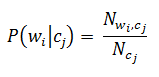Here,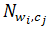is the Number of times word wi appears in documents under class cj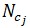is the Count of words appears in all documents that are listed under class cj.
Prior probability
Prior probability is the total probability of a class. That is, how often does this particular class occur in total? This can be calculated as follows;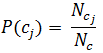Here,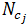is the Total number of documents that are listed under class cj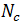is the total number of classes
For the given problem, we need to calculate these probabilities for the test document ‘Chennai Hyderabad’. It goes as follows;
Conditional probability estimation
P(word | class) = P(Chennai|India) = 2/7
[How P(Chennai|India) = 2/7? As per the training data given, only 2 documents (documents 1 and 3) are listed under the class 'India' and have the word 'Chennai'.  hence, 2 in the numerator. There are totally 7 words (2 words in doc 1, 2 in doc 3, and 3 in doc 4) in all the documents under the class 'India' put together. For the remaining conditional probabilities, you do the calculation.]
P(Chennai | England) = 1/6
Prior probability estimation
P(India) = 3/5  [How P(India) = 3/5? As per the training data, out of 5 documents, only 3 are listed under the class 'India'.]
P(England) = 2/5

b) To predict the correct class of the test document ‘Chennai Hyderabad’, we need to find the posterior probability of the test document under each class as follows;
 As per Naïve Bayes, the posterior probability for n features for a class cj is calculated as follows; P(w1, w2, …, wn|cj) = P(cj) * P(w1|cj) * P(w2|cj) * … * P(wn|cj)

P(‘Chennai Hyderabad’ | India) = P(India) * P(Chennai | India) * P(Hyderabad | India)
= 3/5 * 2/7 * 1/7
= 0.6 * 0.286 * 0.143
= 0.0245
P(‘Chennai Hyderabad’ | England) = P(England) * P(Chennai | England) * P(Hyderabad | England)
= 2/5 * 1/6 * 1/6
= 0.4 * 0.167 * 0.167
= 0.0112
After the calculation, we found that P(‘Chennai Hyderabad’ | India) > P(‘Chennai Hyderabad’ | England). Hence, the predicated class of the given document is India.

***********